Home Technical Mathematics Languages Science Social Science Art Literature Arabic Islamic Studies C.R.K History MusicVisual Art Clothing/Textile Home Management Shorthand
 Physics Paper 2, Nov/Dec. 2013
 Questions: 1 2 3 Main
Weakness/Remedies
Strength

Question 1

Question 1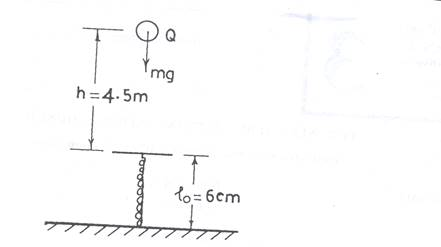An object, Q of mass, m was released from a height and allowed to fall freely unto a vertical spring of natural length,o as shown in the diagram above.  The length,of the compressed spring was recorded.  The height, h of the object above the spring was also recorded.  The procedure was repeated with the object falling from the same height.

The whole procedure was repeated for four other values of h.  In each case,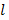was measured twice.

Figure 1(a) shows the heights, hi of the object above the spring while figures 1(b) and 1(c) are scaled diagrams of the spring showing the compressed lengths,i for the first and second readings respectively, where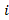= 1,2,3,4 and 5 respectively.

1.  The length AB in figure 1(a) represents the maximum height,hi  =  4.5m, of the object above the spring.  Deduce the scale of the figure and use it to determine and record the values of h2,h3,h4 and h5 respectively.
2. Measure and record the corresponding values offor the two sets.
3. Determine and record the mean value ofin each case.
4. Evaluate e =omean in each case. Also evaluate e2
6. Plot a graph with h on the vertical axis and e2 on the horizontal axis.
7. Determine the slope, s, of the graph.
8. From the graph, deduce the compression that would be produced when the object is dropped from a height of 3.6m.
9. State two precautions that are necessary to ensure accurate results when performing this experiment.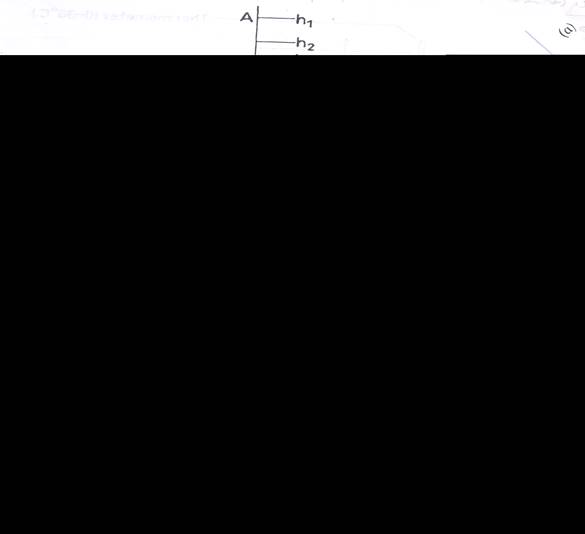(b)       (i)         A spring of force constant 300 Nm-1 is compressed such that
its length shortens by 3.0cm.  Calculate the energy stored in
the spring.

(ii)        The table below shows that loads that hung on a spring
balance caused the pointer to move to the indicated marks.

 Load/N 5 10 F Mark/cm 8 12 30

Calculate the value of F that will move the pointer to the 30 cm mark.

This question was the least popular among the candidates.  Candidates were required to determine the scale factor used in the diagram.  The determination of the scale factor was a major problem to most candidates as they appeared not to know how to go about this.  Out of the few that got the scale right, some could not evaluate the value of e2 to the required degree of 3 significant figure.  Determination of lmean was poorly handled by most responding candidates.  The graph and deductions of e from the graph even when they got e graphically was poorly done.  Many candidates could not state satisfactorily precautions relevant to the experiment.
Part (b)(i) Most candidates were able to calculate the energy stored in the spring
(ii)  This was poorly handled.

_____________________________________________________________________________________________________

Candidates are expected to:

1.       Measure and record AB to at least  1 d. p. and within tolerance of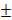0.1 cm
2.       Measure and record four values of h
3.       Measure and recorded  five values of lmean
4.    Evaluate and record five values of e =lo - lmean
5.    Evaluate and record e2
7. Plot a graph with h on the vertical axis and e2 on the horizontal axis using reasonable scales
8. Distinguish between the axes and draw line of best fit
9. Determine the slope of the graph
10. Deduce the value of e for h = 360 cm
11. State any two of the following precautions

1. Parallax error avoided on metre rule
2. Ensured spring is vertical
3. Ensured object is dropped along vertical axis of the spring
4. Noted/ corrected  zero error of metre rule.

1.  Calculate energy stored in a spring using the given data as follows

E = ½ ke2
= ½ x 300 x (0.03)2
= 0.135J

1.  Calculate the value of F when the pointer is the 30 cm mark using the given data as follows:

F = ke
10 - 5    = k (12 - 8)................1
F – 10   = k (30 – 12).............2
Solving simultaneously

F = 32.5N
Graphical method is also acceptable.